# Percentage Quiz for IBPS , SBI , RBI: Quiz – 10

## Percentage Quiz for IBPS , SBI , RBI: Quiz – 10

Percentage is an important topic of the Quantitative Aptitude section of many banking exams such as the IBPS, SBI, and RBI PO and Clerk exams. 1-2 Percentage Questions are generally asked in competitive exams. Thus, candidates must have a clear understanding of the Percentage Questions. So, here we bring to you the Percentage Quiz to help you prepare well. All of the most recent pattern-based questions, as well as Previous Year Percentage Questions from the IBPS, SBI, and RBI PO and Clerk exams, are included in this Percentage Quiz. This Percentage Quiz is free of cost. Each question of this Percentage Quiz comes up with a detailed explanation.

1. A shopkeeper marks the prices at 25% higher than the original price. Due to increase in demand, he further increases the price by 10%. How much % profit will be get?
(a) 32%
(b) 34.5%
(c) 37.5%
(d) 42.5%
(e) None of these2. 5% of income of A is equal to 15% of income of B and 10% of income of B is equal to 20% of income of C. If the income of C is Rs. 2000, what is the total income of A, B and C ?
(a) Rs. 14000
(b) Rs. 16000
(c) Rs. 18000
(d) Rs. 12400
(e) None of these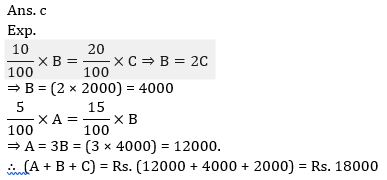3. The number of employees working in a company is increased by 35% and the wages per head are decreased by 35%. What is the percentage decrease in the total wages?4. Suraj loses 13% of his money and after spending 65% of the remaining he is left with Rs 1522.5. How much money did he have in the beginning?
(a) Rs 6000
(b) Rs 6525
(c) Rs 7500
(d) Rs 5225
(e) Rs 5000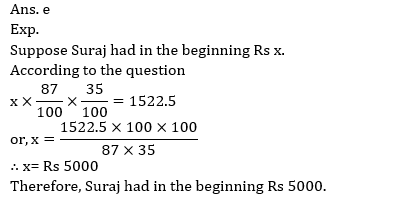5. Akash’s expense is 30% more than Vimal’s and Vimal’s expense is 10% less than Raman’s. If the sum of their expenses is Rs. 6,447, then what would be Akash’s expense?
(a) Rs. 2,200
(b) Rs. 2,457
(c) Rs. 1,890
(d) Rs. 2,100
(e) None of these6. Bina’s monthly income is 90% of Anita’s monthly income. The total of both of their monthly incomes is Mr. Sen’s monthly income. Mr. Sen’s annual income is Rs. 7,75,200. What is Bina’s monthly income?
(a) Rs. 34,000
(b) Rs. 36,000
(c) Rs. 30,600
(d) Rs. 30,000
(e) Rs. 35,000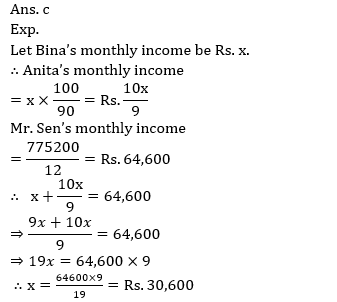7. If the nominator of a fraction is increased by 20% and denominator is reduced by 30%, the new fraction thus formed is 8/21. Find the original fraction
(a) 2/9
(b) 4/9
(c) 5/9
(d) 9/2
(e) None of these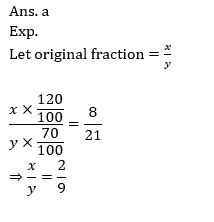8. In an election between 2 candidates, 75% of the voters cast their votes, out of which 2% votes were declared invalid. A candidate got 18522 votes which were 75% of the valid votes. The total number of voters enrolled in the election was:
(a)36000
(b)36400
(c)33600
(d)34800
(e) 38500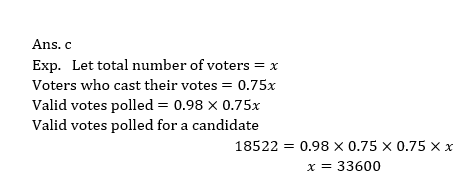9. In an examination paper of 5 questions, 5% of the candidates answered all of them and 5% none. Of the rest 25% answered only 1 question and 20% answered only 4. If 49/2% of the entire candidates answered only 2 questions and 200 answered only 3, how many candidates appeared at the examination?
(a) 1000
(b) 1200
(c) 700
(d) 800
(e) None of these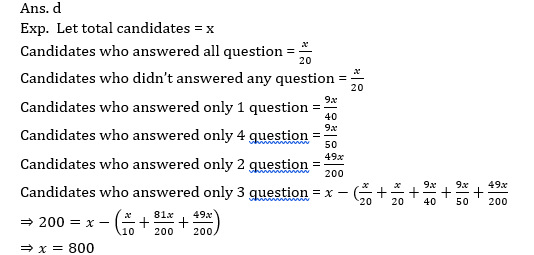10. In a test Manjit got 232 marks which were 56 more than that of the Saurabh’s marks. Supriya got 40% marks out of the total marks of the test which were 24 marks more than the Saurabh’s marks. What percentage of marks did Saurabh get ?
(a) 35
(b) 35.2
(c) 42
(d) 36.2
(e)37.6#### Attempt Quantitative Aptitude Topic Wise Online Test Series

Recommended PDF’s for:

#### Most important PDF’s for Bank, SSC, Railway and Other Government Exam : Download PDF Now

AATMA-NIRBHAR Series- Static GK/Awareness Practice Ebook PDF Get PDF here
The Banking Awareness 500 MCQs E-book| Bilingual (Hindi + English) Get PDF here
AATMA-NIRBHAR Series- Banking Awareness Practice Ebook PDF Get PDF here
Computer Awareness Capsule 2.O Get PDF here
AATMA-NIRBHAR Series Quantitative Aptitude Topic-Wise PDF Get PDF here
AATMA-NIRBHAR Series Reasoning Topic-Wise PDF Get PDF Here
Memory Based Puzzle E-book | 2016-19 Exams Covered Get PDF here
Caselet Data Interpretation 200 Questions Get PDF here
Puzzle & Seating Arrangement E-Book for BANK PO MAINS (Vol-1) Get PDF here
ARITHMETIC DATA INTERPRETATION 2.O E-book Get PDF here
3